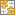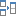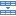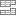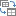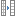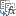# Excel dynamic arrays, functions and formulas

Due to the revolutionary update in the Excel 365 calculation engine, array formulas become very straightforward and understandable for everyone, not just for super users. The tutorial explains the concept of new Excel dynamic arrays and shows how they can make your worksheets more efficient and a lot easier to set up.

Excel array formulas have always been considered a prerogative of gurus and formula experts. If someone says to you, "This can be done with an array formula", an immediate reaction of many users is "Oh, isn't there another way?".

The introduction of dynamic arrays is a long awaited and most welcome change. Due to their ability to work with multiple values in a simple manner, without any tricks and quirks, dynamic array formulas are something that every Excel user can understand and enjoy creating.

## Excel dynamic arrays

Dynamic Arrays are resizable arrays that calculate automatically and return values into multiple cells based on a formula entered in a single cell.

Through over 30 years of history, Microsoft Excel has undergone many changes, but one thing remained constant - one formula, one cell. Even with traditional array formulas, it was necessary to enter a formula into each cell where you want a result to appear. With dynamic arrays, this rule is no longer true. Now, any formula that returns an array of values automatically spills into neighboring cells, without you having to press Ctrl + Shift + Enter or do any other moves. In other words, operating dynamic arrays becomes as easy as working with a single cell.

Let me illustrate the concept with a very basic example. Supposing, you need to multiply two groups of numbers, for example, to calculate different percentages.

In pre-dynamic versions of Excel, the below formula would work for the first cell only, unless you enter it in multiple cells and press Ctrl + Shift + Enter to explicitly make it an array formula:

`=A3:A5*B2:D2`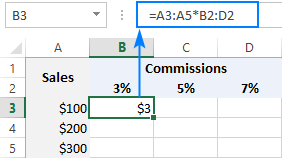Now, see what happens when the same formula is used in Excel 365. You type it in just one cell (B3 in our case), press the Enter key… and have the whole rage filled with the results at once: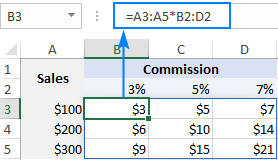Filling multiple cells with a single formula is called spilling, and the populated range of cells is called the spill range.

An important thing to note is that the recent update is not just a new way of handling arrays in Excel. In fact, this is a groundbreaking change to the entire calculation engine. With dynamic arrays, a bunch of new functions have been added to the Excel Function Library and the existing ones started to work faster and more effectively. Eventually, new dynamic arrays are supposed to completely replace the old-fashioned array formulas that are input with the Ctrl + Shift + Enter shortcut.

## Excel dynamic arrays availability

Dynamic arrays were introduced at the Microsoft Ignite Conference in 2018, released to Office 365 subscribers in January 2020, and are only available with Microsoft 365 subscriptions.

• Excel 365 for Windows
• Excel 365 for Mac
• Excel 365 for Apple
• Excel 365 for Android
• Excel 365 for Windows Mobile

Please note that Excel Online doesn't support dynamic arrays.

## Excel dynamic array functions

As part of the new functionality, 6 new functions were introduced in Excel 365 that handle arrays natively and output data into a range of cells. The output is always dynamic - when any change occurs in the source data, the results update automatically. Hence the group name - dynamic array functions.

These new functions easily cope with a number of tasks that are traditionally considered hard nuts to crack. For example, they can remove duplicates, extract and count unique values, filter out blanks, generate random integers and decimal numbers, sort in ascending or descending order, and a lot more.

Below you will find a brief description of what each function does as well as the links to in-depth tutorials:

1. UNIQUE - extracts unique items from a range of cells.
2. FILTER - filters data based in the criteria you define.
3. SORT - sorts a range of cells by a specified column.
4. SORTBY - sorts a range of cells by another range or array.
5. RANDARRAY - generates an array of random numbers.
6. SEQUENCE - generates a list of sequential numbers.

Additionally, there are two modern replacements of the popular Excel functions, which are not officially in the group, but leverage all the advantages of dynamic arrays:

XLOOKUP - is a more powerful successor of VLOOKUP, HLOOKUP and LOOKUP that can look up both in columns and rows and return multiple values.

XMATCH - is a more versatile successor of the MATCH function that can perform vertical and horizontal lookups and return a relative position of the specified item.

## Excel dynamic array formulas

In the latest versions of Excel 365, the dynamic array behavior is deeply integrated and becomes native for all functions, even those that were not originally designed to work with arrays. To put it simply, for any formula that returns more than one value, Excel automatically creates a resizable range into which the results are output. Due to this ability, the existing functions can now perform magic!

The below examples show new dynamic array formulas in action as well as the effect of dynamic arrays on existing functions.

### Example 1. New dynamic array function

This example demonstrates how much faster and simpler a solution can be accomplished with Excel dynamic array functions.

To extract a list of unique values from a column, you'd traditionally use a complex CSE formula like this one. In Excel 365, all you need is a UNIQUE formula in its basic form:

`=UNIQUE(B2:B10)`

You enter the formula in any empty cell and hit Enter. Excel immediately extracts all different values in the list and outputs them into a range of cells beginning from the cell where you entered the formula (D2 in our case). When the source data changes, the results are recalculated and updated automatically.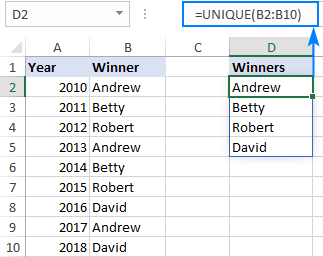### Example 2. Combining several dynamic array functions in one formula

If there is no way to accomplish a task with one function, chain a few ones together! For example, to filter data based on condition and arrange the results alphabetically, wrap the SORT function around FILTER like this:

`=SORT(FILTER(A2:C13, B2:B13=F1, "No results"))`

Where A2:C13 are the source data, B2:B13 are the values to check, and F1 is the criterion.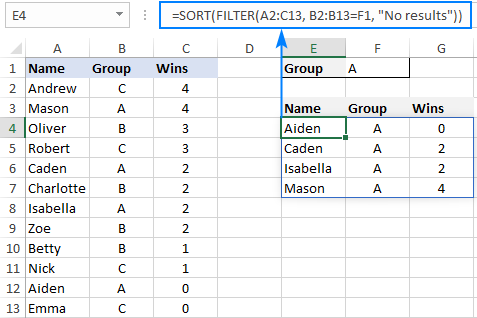### Example 3. Using new dynamic array functions together with existing ones

As the new calculation engine implemented in Excel 365 can easily turn conventional formulas into arrays, there's nothing that would prevent you from combining new and old functions together.

For instance, to count how many unique values there are in a certain range, nest the dynamic array UNIQUE function into the good old COUNTA:

`=COUNTA(UNIQUE(B2:B10))`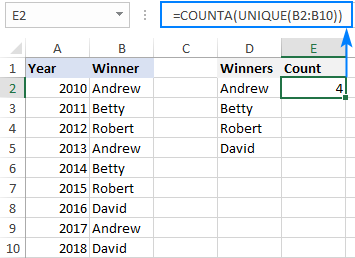### Example 4. Existing functions support dynamic arrays

If you supply a range of cells to the TRIM function in an older version such as Excel 2016 or Excel 2019, it will return a single result for the first cell:

`=TRIM(A2:A6)`

In dynamic Excel, the same formula processes all of the cells and returns multiple results, as shown below: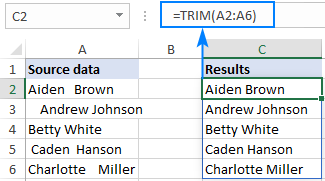### Example 5. VLOOKUP formula to return multiple values

As everyone knows, the VLOOKUP function is designed to return a single value based on the column index that you specify. In Excel 365, however, you can supply an array of column numbers to return matches from several columns:

`=VLOOKUP(F1, A2:C6, {1,2,3}, FALSE)`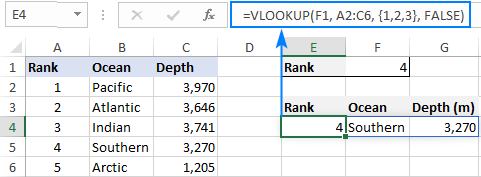### Example 6. TRANSPOSE formula made easy

In earlier Excel versions, the syntax of the TRANSPOSE function left no room for mistakes. To rotate data in your worksheet, you needed to count the original columns and rows, select the same number of empty cells but change the orientation (a mind-boggling operation in huge worksheets!), type a TRANSPOSE formula in the selected range, and press Ctrl + Shift + Enter to complete it correctly. Phew!

In dynamic Excel, you just enter the formula in the leftmost cell of the output range and press Enter:

`=TRANSPOSE(A1:B10)`

Done!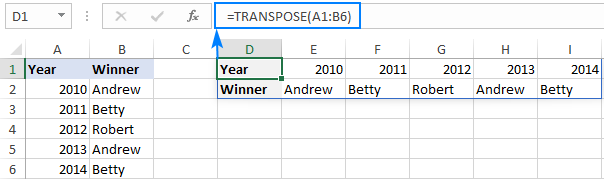## Spill range - one formula, multiple cells

The spill range is a range of cells that contains the results returned by a dynamic array formula.

When any cell in the spill range is selected, the blue border appears to show that everything inside it is calculated by the formula in the top left cell. If you delete the formula in the first cell, all the results will be gone.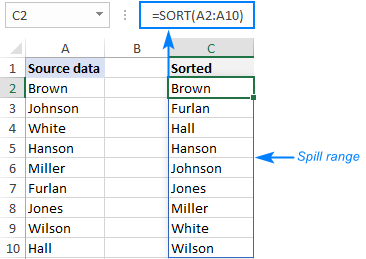The spill range is a really great thing that makes the lives of Excel users a lot easier. Previously, with CSE array formulas, we had to guess at how many cells to copy them to. Now, you just enter the formula in the first cell and let Excel take care of the rest.

Although the spill range behavior is fully dynamic (when the original data changes, the spill range expands or contracts correspondingly), it does not update when new entries are added outside the referred range. For example, our SORT formula processed data in A2:A10. In case a new value is input in A11, it won't be included in the results unless you change the range reference in the formula. If you want such changes to be reflected on the fly, then convert your source range to an Excel table and use structured references in your formulas. Unlike ranges, Excel tables expand automatically to include newly added rows. The same effect can be achieved with a dynamic named range.

Note. If some other data is blocking the spill range, a #SPILL error occurs. Once the obstructing data is removed, the error will be gone.

## Spill range reference (# symbol)

To refer to the spill range, put a hash tag or pound symbol (#) after the address of the upper left cell in the range.

For example, to reference the results of a dynamic array formula in C2, type:

`=C2#`

To refer to the same spill range from another sheet, include the sheet's name in the reference:

`=Sheet1!C2#`

This is the same as referencing the entire range (=C2:C8), but unlike a regular reference, the spill ref automatically reflects changes in the range size. This behavior is especially useful when you are "feeding" the spill range to another function, either dynamic or conventional one. You can even use it for named ranges and data validation.

For example, to find how many random numbers are generated by the RANDARRAY formula in C2, supply the spill range reference to the COUNTA function:

`=COUNTA(C2#)`

To add up the values in the spill range, use:

`=SUM(C2#)`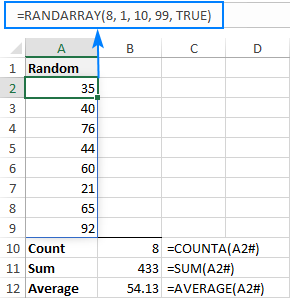Tip. To quickly refer to a spill range, simply select all the cells inside the blue box using the mouse. Excel will create the spill ref for you automatically.

## Implicit intersection and @ character

In dynamic array Excel, there is one more significant change in the formula language - the introduction of the @ character, known as the implicit intersection operator.

In Microsoft Excel, implicit intersection is a formula behavior that reduces many values to a single value. In old Excel, a cell could only contain a single value, so that was the default behavior and no special operator was needed for it.

In Excel 365, all formulas are regarded as array formulas by default. The implicit intersection operator is used to prevent the array behavior if you do not want it in a specific formula. In other words, if you wish the formula to return just one value, put @ before the function's name, and it will behave like a non-array formula in traditional Excel.

To see how it works in practice, please take a look at the screenshot below.

In C2, there's a dynamic array formula that spills results in many cells:

`=UNIQUE(A2:A9)`

In E2, the function is prefixed with the @ character that invokes implicit intersection. As the result, only the first unique value is returned:

`=@UNIQUE(A2:A9)`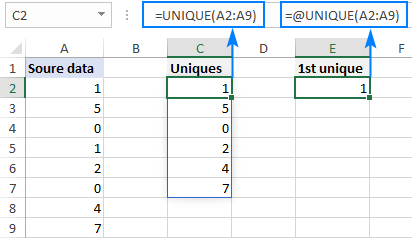When opening a worksheet created in an older version of Excel, you may notice the @ symbol added automatically to formulas that can potentially return multiple values. This is done to force the formula to behave the same way as it did in the original Excel version. Depending on the formula, removing @ will either spill the results to multiple cells or not have any noticeable effect at all.

Note. Initially, Microsoft introduced the SINGLE function for the same purpose, but later on replaced it with the @ operator.

## Advantages of Excel dynamic arrays

Undoubtedly, dynamic arrays is one of the best Excel enhancements in years. Like any new feature, it has its strong and its weak points. Luckily for us, the strong points of new Excel dynamic array formulas are overwhelming!

### Simple and more powerful

Dynamic arrays make it possible to create more powerful formulas in a much simpler way. Here are a couple of examples:

### Native for all formulas

In dynamic Excel, you do not need to bother which functions support arrays and which do not. If a formula can return multiple values, it will do so by default. This also applies to arithmetic operations and legacy functions as demonstrated in this example.

### Nesting dynamic array functions

To work out solutions for more complex tasks, you are free to combine new Excel dynamic array functions or use them together with old ones like shown here and here.

### Relative and absolute references are less important

Thanks to the "one formula, many values" approach, there is no need to lock ranges with the \$ sign since, technically, the formula is in just one cell. So, for the most part, it does not really matter whether to use absolute, relative or mixed cell references (which has always been a source of confusion for inexperienced users) - a dynamic array formula will produce correct results anyway!

## Limitations of dynamic arrays

New dynamic arrays are great, but as with any new feature there are a few caveats and considerations that you should be aware of.

### Results cannot be sorted in the usual way

The spill range returned by a dynamic array formula cannot be sorted by using Excel's Sort feature. Any such attempt will result in the "You cannot change part of an array" error. To arrange the results from smallest to largest or vice versa, wrap your current formula in the SORT function. For example, this is how you can filter and sort at a time.

### Cannot delete any value in spill range

None of the values in a spill range can be deleted because of the same reason: you cannot change part of an array. This behavior is expected and logical. Traditional CSE array formulas also work this way.

### Results cannot be put in a table

And this feature (or bug?) is quite unexpected. If you try to convert a spill range to an Excel table, Excel will do so. But instead of the results, you will only see a #SPILL! error. Hopefully, they will fix it in one of the next builds.

### Do not work with Excel Power Query

The results of dynamic array formulas cannot be loaded into Power Query. Say, if you try to merge two or more spill ranges together using Power Query, this won't work.

## Dynamic arrays vs. traditional CSE array formulas

With the introduction of dynamic arrays, we can talk about two types of Excel:

1. Dynamic Excel that fully supports dynamic arrays, functions and formulas. Currently it's only Excel 365.
2. Legacy Excel, aka traditional or pre-dynamic Excel, where only Ctrl + Shift + Enter array formulas are supported. It's Excel 2019, Excel 2016, Excel 2013 and earlier versions.

It goes without saying that dynamic arrays are superior to CSE array formulas in all respects. Although the traditional array formulas are retained in Excel 365 for compatibility reasons, from now on it is recommended to use the new ones.

Here are the most essential differences:

• A dynamic array formula is entered in one cell and completed with a regular Enter keystroke. To complete an old-fashioned array formula, you need to press Ctrl + Shift + Enter.
• New array formulas spill to many cells automatically. CSE formulas must be copied to a range of cells to return multiple results.
• The output of dynamic array formulas automatically resizes as the data in the source range changes. CSE formulas truncate the output if the return area is too small and return errors in extra cells if the return area is too large.
• A dynamic array formula can be easily edited in a single cell. To modify a CSE formula, you need to select and edit the whole range.
• It is not possible to delete and insert rows in a CSE formula range - you need to delete all existing formulas first. With dynamic arrays, row insertion or deletion is not a problem.

## Backward compatibility: dynamic arrays in legacy Excel

When you open a workbook containing a dynamic array formula in old Excel, it is automatically converted to a conventional array formula enclosed in {curly braces}. When you open the worksheet again in Excel 365, the curly braces will be removed.

In legacy Excel, the new dynamic array functions and spill range references get prefixed with _xlfn to indicate that this functionality is not supported. A spill range ref sign (#) is replaced with the ANCHORARRAY function.

For example, here's how a UNIQUE formula appears in Excel 2013: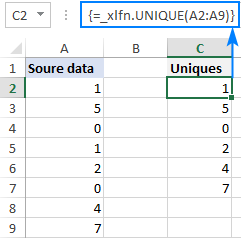Most dynamic array formulas (but not all!) will keep displaying their results in legacy Excel until you make any changes to them. Editing a formula immediately breaks it and displays one or more #NAME? error values.

## Excel dynamic array formulas not working

Depending on the function, different errors may occur if you use an incorrect syntax or invalid arguments. Below are the 3 most common errors that you may run into with any dynamic array formula.

### #SPILL! error

When a dynamic array returns multiple results, but something is blocking the spill range, a #SPILL! error occurs.

To fix the error, you just need to clear or delete any cells in the spill range that are not completely blank. To quickly spot all the cells that get in the way, click the error indicator, and then click Select Obstructing Cells.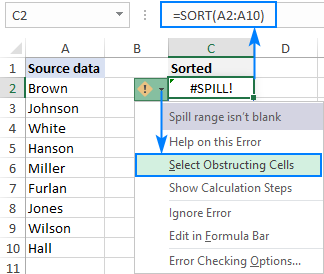### #REF! error

Because of the limited support for external references between workbooks, dynamic arrays require both files to be open. If the source workbook is closed, a #REF! error is displayed.

### #NAME? error

A #NAME? error occurs if you attempt to use a dynamic array function in an older version of Excel. Please remember that the new functions are only available in Excel 365.

If this error appears in Excel 365, double-check the function's name in the problematic cell. Chances are it is mistyped :)

That's how to use dynamic arrays in Excel. Hopefully, you will love this fantastic new functionality! Anyway, I thank you for reading and hope to see you on our blog next week!

### 2 responses to "Excel dynamic arrays, functions and formulas"

1.Matthew says:

This was a great article, thank you very much.

2.David says:

Is there any way to use dynamic array functions to reference multiple ranges, for example:
=unique({A1:A6,D1:D6})

Ultimate Suite for Microsoft Excel